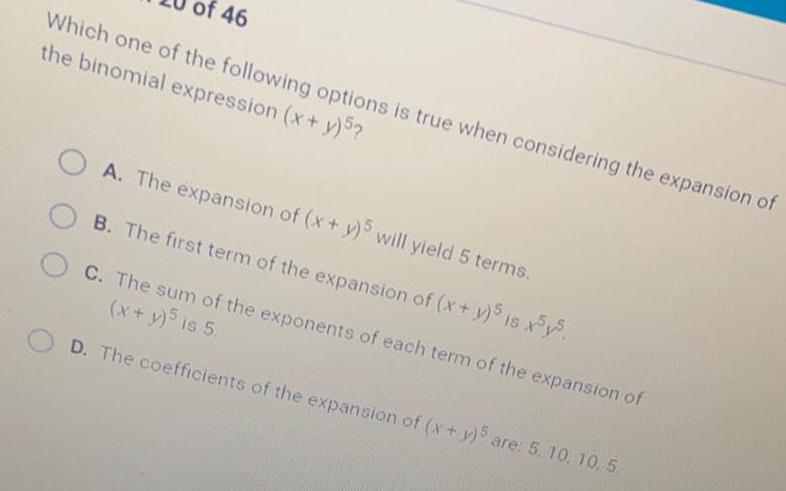Question:

# Which one of the following options is true when considering

Last updated: 7/21/2022Which one of the following options is true when considering the expansion of the binomial expression (x + y)5? A. The expansion of (x + y)5 will yield 5 terms. B. The first term of the expansion of (x + y)5 is x5y5. C. The sum of the exponents of each term of the expansion of (x + y)5 is 5. D. The coefficients of the expansion of (x + y)5 are: 5, 10, 10, 5.